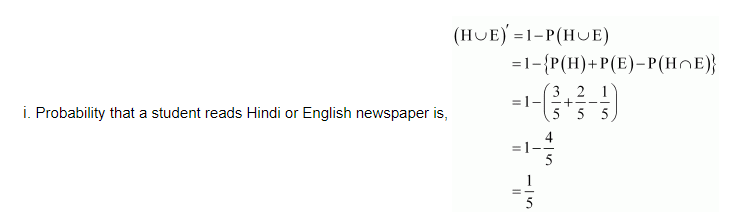# In a hostel, 60% of the students read Hindi newspaper,

Question:

In a hostel, 60% of the students read Hindi newspaper, 40% read English newspaper and 20% read both Hindi and English news papers. A student is selected at random.

(a) Find the probability that she reads neither Hindi nor English news papers.

(b) If she reads Hindi news paper, find the probability that she reads English news paper.

(c) If she reads English news paper, find the probability that she reads Hindi news paper.

Solution:

Let H denote the students who read Hindi newspaper and E denote the students who read English newspaper. It is given that,

$\mathrm{P}(\mathrm{H})=60 \%=\frac{6}{10}=\frac{3}{5}$

$\mathrm{P}(\mathrm{E})=40 \%=\frac{40}{100}=\frac{2}{5}$

$\mathrm{P}(\mathrm{H} \cap \mathrm{E})=20 \%=\frac{20}{100}=\frac{1}{5}$(ii) Probability that a randomly chosen student reads English newspaper, if she reads Hindi news paper, is given by P (E|H

$P(E \mid H)=\frac{P(E \cap H)}{P(H)}$

$=\frac{\frac{1}{5}}{\frac{3}{5}}$

$=\frac{1}{3}$

(iii) Probability that a randomly chosen student reads Hindi newspaper, if she reads English newspaper, is given by P (H|E).

$P(H \mid E)=\frac{P(H \cap E)}{P(E)}$

$=\frac{1}{\frac{5}{2}}$

$=\frac{1}{5}$